Home » Comprehensive Guide on t-SNE algorithm with implementation in R & Python

# Comprehensive Guide on t-SNE algorithm with implementation in R & Python

## Introduction

Imagine you get a dataset with hundreds of features (variables) and have little understanding about the domain the data belongs to. You are expected to identify hidden patterns in the data, explore and analyze the dataset. And not just that, you have to find out if there is a pattern in the data – is it signal or is it just noise?

Does that thought make you uncomfortable? It made my hands sweat when I came across this situation for the first time. Do you wonder how to explore a multidimensional dataset? It is one of the frequently asked question by many data scientists. In this article, I will take you through a very powerful way to exactly do this.

By now, some of you would be screaming “I’ll use PCA for dimensionality reduction and visualization”. Well, you are right! PCA is definitely a good choice for dimensionality reduction and visualization for datasets with a large number of features. But, what if you could use something more advanced than PCA? (If you don’t know PCA, I would strongly recommend to read this article first)

What if you could easily search for a pattern in non-linear style? In this article, I will tell you about a new algorithm called t-SNE (2008), which is much more effective than PCA (1933). I will take you through the basics of t-SNE algorithm first and then will walk you through why t-SNE is a good fit for dimensionality reduction algorithms.

You will also, get hands-on knowledge for using t-SNE in both R and Python.## Table of Content

1. What is t-SNE?
2. What is dimensionality reduction?
3. How does t-SNE fit in the dimensionality reduction algorithm space
4. Algorithmic details of t-SNE
• Algorithm
• Time and Space Complexity
5. What does t-SNE actually do?
6. Use cases
7.  t-SNE compared to other dimensionality reduction algorithm
8. Example Implementations
• In R
• Hyper parameter tuning
• Code
• Implementation Time
• Interpreting Results
• In Python
• Hyper parameter tuning
• Code
• Implementation Time
9. Where and when to use
• Data Scientist
• Machine Learning Competition Enthusiast
• Student
10. Common fallacies

## 1. What is t-SNE?

(t-SNE) t-Distributed Stochastic Neighbor Embedding is a non-linear dimensionality reduction algorithm used for exploring high-dimensional data. It maps multi-dimensional data to two or more dimensions suitable for human observation. With help of the t-SNE algorithms, you may have to plot fewer exploratory data analysis plots next time you work with high dimensional data.## 2. What is dimensionality reduction?

In order to understand how t-SNE works, let’s first understand what is dimensionality reduction?

Well, in simple terms, dimensionality reduction is the technique of representing multi-dimensional data (data with multiple features having a correlation with each other) in 2 or 3 dimensions.

Some of you might question why do we need Dimensionality Reduction when we can plot the data using scatter plots, histograms & boxplots and make sense of the pattern in data using descriptive statistics.

Well, even if you can understand the patterns in data and present it on simple charts, it is still difficult for anyone without statistics background to make sense of it. Also, if you have hundreds of features, you have to study thousands of charts before you can make sense of this data. (Read more about dimensionality reduction here)

With the help of dimensionality reduction algorithm, you will be able to present the data explicitly.

## 3. How does t-SNE fit in the dimensionality reduction algorithm space?

Now that you have an understanding of what is dimensionality reduction, let’s look at how we can use t-SNE algorithm for reducing dimensions.

Following are a few dimensionality reduction algorithms that you can check out:

1. PCA (linear)
2. t-SNE (non-parametric/ nonlinear)
3. Sammon mapping (nonlinear)
4. Isomap (nonlinear)
5. LLE (nonlinear)
6. CCA (nonlinear)
7. SNE (nonlinear)
8. MVU (nonlinear)
9. Laplacian Eigenmaps (nonlinear)

The good news is that you need to study only two of the algorithms mentioned above to effectively visualize data in lower dimensions – PCA and t-SNE.

### Limitations of PCA

PCA is a linear algorithm. It will not be able to interpret complex polynomial relationship between features. On the other hand, t-SNE is based on probability distributions with random walk on neighborhood graphs to find the structure within the data.

A major problem with, linear dimensionality reduction algorithms is that they concentrate on placing dissimilar data points far apart in a lower dimension representation. But in order to represent high dimension data on low dimension, non-linear manifold, it is important that similar datapoints must be represented close together, which is not what linear dimensionality reduction algorithms do.

Now, you have a brief understanding of what PCA endeavors to do.

Local approaches seek to map nearby points on the manifold to nearby points in the low-dimensional representation. Global approaches on the other hand attempt to preserve geometry at all scales, i.e mapping nearby points to nearby points and far away points to far away points

It is important to know that most of the nonlinear techniques other than t-SNE are not capable of retaining both the local and global structure of the data at the same time.

## 4. Algorithmic details of t-SNE (optional read)

This section is for the people interested in understanding the algorithm in depth. You can safely skip this section if you do not want to go through the math in detail.

Let’s understand why you should know about t-SNE and the algorithmic details of t-SNE.  t-SNE is an improvement on the Stochastic Neighbor Embedding (SNE) algorithm.

### 4.1 Algorithm

#### Step 1

Stochastic Neighbor Embedding (SNE) starts by converting the high-dimensional Euclidean distances between data points into conditional probabilities that represent similarities. The similarity of datapoint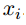to datapoint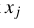is the conditional probability,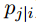,would pickas its neighbor if neighbors were picked in proportion to their probability density under a Gaussian centered atFor nearby datapoints,is relatively high, whereas for widely separated datapoints,will be almost infinitesimal (for reasonable values of the variance of the Gaussian,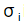). Mathematically, the conditional probabilityis given by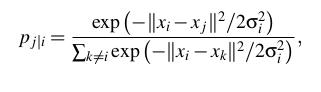whereis the variance of the Gaussian that is centered on datapointIf you are not interested in the math, think about it in this way, the algorithm starts by converting the shortest distance (a straight line) between the points into probability of similarity of points. Where, the similarity between points is: the conditional probability thatwould pickas its neighbor if neighbors were picked in proportion to their probability density under a Gaussian (normal distribution) centered at.

#### Step 2

For the low-dimensional counterparts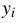and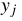of the high-dimensional datapointsandit is possible to compute a similar conditional probability, which we denote by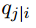.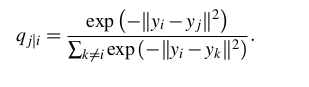Note that, pi|i and pj|j are set to zero as we only want to model pair wise similarity.

In simple terms step 1 and step2 calculate the conditional probability of similarity between a pair of points in

1. High dimensional space
2. In low dimensional space

For the sake of simplicity, try to understand this in detail.

Let us map 3D space to 2D space. What step1 and step2 are doing is calculating the probability of similarity of points in 3D space and calculating the probability of similarity of points in the corresponding 2D space.

Logically, the conditional probabilitiesandmust be equal for a perfect representation of the similarity of the datapoints in the different dimensional spaces, i.e the difference betweenandmust be zero for the perfect replication of the plot in high and low dimensions.

By this logic SNE attempts to minimize this difference of conditional probability.

#### Step 3

Now here is the difference between the SNE and t-SNE algorithms.

To measure the minimization of sum of difference of conditional probability SNE minimizes the sum of Kullback-Leibler divergences overall data points using a gradient descent method. We must know that KL divergences are asymmetric in nature.

In other words, the SNE cost function focuses on retaining the local structure of the data in the map (for reasonable values of the variance of the Gaussian in the high-dimensional space,).

Additionally, it is very difficult (computationally inefficient) to optimize this cost function.

So t-SNE also tries to minimize the sum of the difference in conditional probabilities. But it does that by using the symmetric version of the SNE cost function, with simple gradients. Also, t-SNE employs a heavy-tailed distribution in the low-dimensional space to alleviate both the crowding problem (the area of the two-dimensional map that is available to accommodate moderately distant data points will not be nearly large enough compared with the area available to accommodate nearby data points)  and the optimization problems of SNE.

#### Step 4

If we see the equation to calculate the conditional probability, we have left out the variance from the discussion as of now. The remaining parameter to be selected is the varianceof the student’s t-distribution that is centered over each high-dimensional datapoint. It is not likely that there is a single value ofthat is optimal for all data points in the data set because the density of the data is likely to vary. In dense regions, a smaller value ofis usually more appropriate than in sparser regions. Any particular value ofinduces a probability distribution,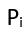, over all of the other data points. This distribution has an

This distribution has an entropy which increases asincreases. t-SNE performs a binary search for the value ofthat produces awith a fixed perplexity that is specified bythe user. The perplexity is defined as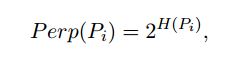where H() is the Shannon entropy ofmeasured in bits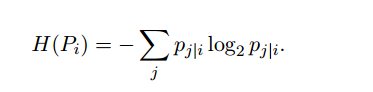The perplexity can be interpreted as a smooth measure of the effective number of neighbors. The performance of SNE is fairly robust to changes in the perplexity, and typical values are between 5 and 50.

The minimization of the cost function is performed using gradient decent. And physically, the gradient may be interpreted as the resultant force created by a set of springs between the map pointand all other map points. All springs exert a force along the direction (–). The spring betweenandrepels or attracts the map points depending on whether the distance between the two in the map is too small or too large to represent the similarities between the two high-dimensional datapoints. The force exerted by the spring betweenandis proportional to its length, and also proportional to its stiffness, which is the mismatch (pj|i – qj|i + p i| j − q i| j ) between the pairwise similarities of the data points and the map points.-

### 4.2 Time and Space Complexity

Now that we have understood the algorithm, it is time to analyze its performance. As you might have observed, that the algorithm computes pairwise conditional probabilities and tries to minimize the sum of the difference of the probabilities in higher and lower dimensions. This involves a lot of calculations and computations. So the algorithm is quite heavy on the system resources.

t-SNE has a quadratic time and space complexity in the number of data points. This makes it particularly slow and resource draining while applying it to data sets comprising of more than 10,000 observations.

## 5. What does t-SNE actually do?

After we have looked into the mathematical description of how does the algorithms works, to sum up, what we have learned above. Here is a brief explanation of how t-SNE works.

It’s quite simple actually, t-SNE a non-linear dimensionality reduction algorithm finds patterns in the data by identifying observed clusters based on similarity of data points with multiple features. But it is not a clustering algorithm it is a dimensionality reduction algorithm. This is because it maps the multi-dimensional data to a lower dimensional space, the input features are no longer identifiable. Thus you cannot make any inference based only on the output of t-SNE. So essentially it is mainly a data exploration and visualization technique.

But t-SNE can be used in the process of classification and clustering by using its output as the input feature for other classification algorithms.

## 6. Use cases

You may ask, what are the use cases of such an algorithm. t-SNE can be used on almost all high dimensional data sets. But it is extensively applied in Image processing, NLP, genomic data and speech processing. It has been utilized for improving the analysis of brain and heart scans. Below are a few examples:

### 6.1 Facial Expression Recognition

A lot of progress has been made on FER and many algorithms like PCA have been studied for FER. But, FER still remains a challenge due to the difficulties of dimension reduction and classification. t-Stochastic Neighbor Embedding (t-SNE) is used for reducing the high-dimensional data into a relatively low-dimensional subspace and then using other algorithms like AdaBoostM2, Random Forests, Logistic Regression, NNs and others as multi-classifier for the expression classification.

In one such attempt for facial recognition based on the Japanese Female Facial Expression (JAFFE) database with t-SNE and AdaBoostM2. Experimental results showed that the proposed new algorithm applied to FER gained the better performance compared with those traditional algorithms, such as PCA, LDA, LLE and SNE.

The flowchart for implementing such a combination on the data could be as follows:

Preprocessing → normalization → t-SNE→ classification algorithm

PCA      LDA    LLE     SNE    t-SNE

SVM               73.5%  74.3%  84.7%  89.6%  90.3%

AdaboostM2   75.4%  75.9%  87.7%  90.6%  94.5%

### 6.2 Identifying Tumor subpopulations (Medical Imaging)

Mass spectrometry imaging (MSI) is a technology that simultaneously provides the spatial distribution for hundreds of biomolecules directly from tissue. Spatially mapped t-distributed stochastic neighbor embedding (t-SNE), a nonlinear visualization of the data that is able to better resolve the biomolecular intratumor heterogeneity.

In an unbiased manner, t-SNE can uncover tumor subpopulations that are statistically linked to patient survival in gastric cancer and metastasis status in primary tumors of breast cancer. Survival analysis performed on each t-SNE clusters will provide significantly useful results.

### 6.3 Text comparison using wordvec

Word vector representations capture many linguistic properties such as gender, tense, plurality and even semantic concepts like “capital city of”. Using dimensionality reduction, a 2D map can be computed where semantically similar words are close to each other. This combination of techniques can be used to provide a bird’s-eye view of different text sources, including text summaries and their source material. This enables users to explore a text source like a geographical map.

## 7. t-SNE compared to other dimensionality reduction algorithms

While comparing the performance of t-SNE with other algorithms, we will compare t-SNE with other algorithms based on the achieved accuracy rather than the time and resource requirements with relation to accuracy.

t-SNE outputs provide better results than PCA and other linear dimensionality reduction models. This is because a linear method such as classical scaling is not good at modeling curved manifolds. It focuses on preserving the distances between widely separated data points rather than on preserving the distances between nearby data points.

The Gaussian kernel employed in the high-dimensional space by t-SNE defines a soft border between the local and global structure of the data. And for pairs of data points that are close together relative to the standard deviation of the Gaussian, the importance of modeling their separations is almost independent of the magnitudes of those separations. Moreover, t-SNE determines the local neighborhood size for each datapoint separately based on the local density of the data (by forcing each conditional probability distribution to have the same perplexity). This is because the algorithm defines a soft border between the local and global structure of the data.  And unlike other non-linear dimensionality reduction algorithms, it performs better than any of them.

## 8. Example Implementations

Let’s implement the t-SNE algorithm on MNIST handwritten digit database. This is one of the most explored dataset for image processing.

### 1. In R

The “Rtsne” package has an implementation of t-SNE in R. The “Rtsne” package can be installed in R using the following command typed in the R console:

`install.packages(“Rtsne”)`
• #### Hyper parameter tuning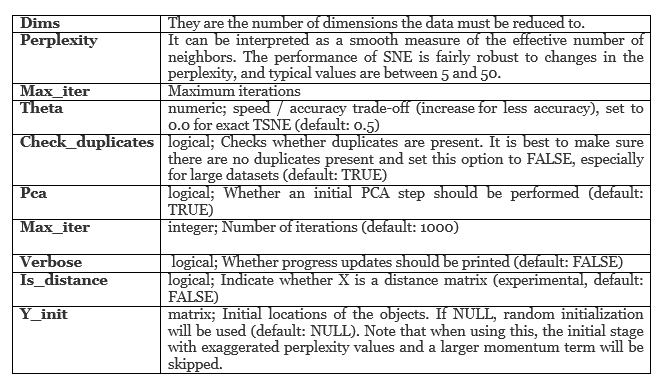• #### Code

```## calling the installed package
library(Rtsne)
## Curating the database for analysis with both t-SNE and PCA
Labels<-train\$label
train\$label<-as.factor(train\$label)
## for plotting
colors = rainbow(length(unique(train\$label)))
names(colors) = unique(train\$label)

## Executing the algorithm on curated data
tsne <- Rtsne(train[,-1], dims = 2, perplexity=30, verbose=TRUE, max_iter = 500)
exeTimeTsne<- system.time(Rtsne(train[,-1], dims = 2, perplexity=30, verbose=TRUE, max_iter = 500))

## Plotting
plot(tsne\$Y, t='n', main="tsne")
text(tsne\$Y, labels=train\$label, col=colors[train\$label])```

• #### Implementation Time

```exeTimeTsne
user       system  elapsed
118.037   0.000  118.006

exectutiontimePCA
user     system   elapsed
11.259   0.012     11.360```

As can be seen t-SNE takes considerably longer time to execute on the same sample size of data than PCA.

• #### Interpreting Results

The plots can be used for exploratory analysis. The output x & y co-ordinates and as well as cost can be used as features in classification algorithms.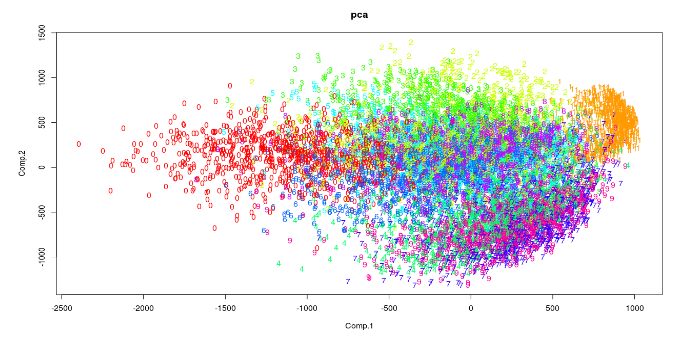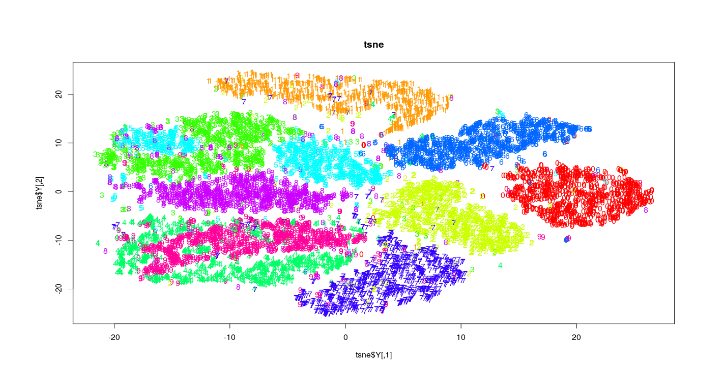### 2. In Python

An important thing to note is that the “pip install tsne” produces an error. Installing “tsne” package is not recommended. t-SNE algorithm can be accessed from sklearn package.

• #### Hyper parameter tuning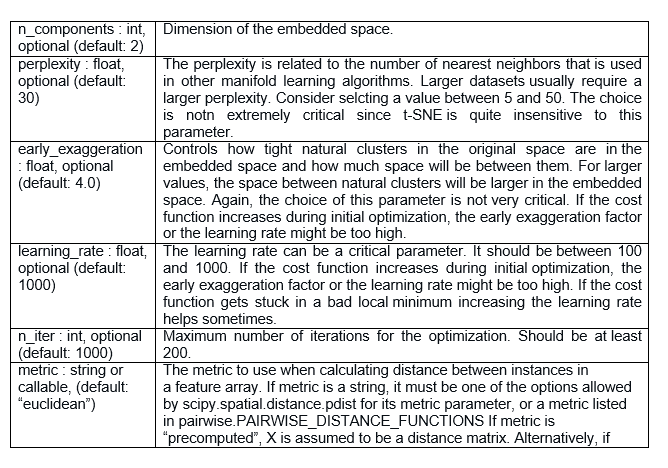• #### Code

The following code is taken from the sklearn examples on the sklearn website.

```## importing the required packages
from time import time
import numpy as np
import matplotlib.pyplot as plt
from matplotlib import offsetbox
from sklearn import (manifold, datasets, decomposition, ensemble,
discriminant_analysis, random_projection)
X = digits.data
y = digits.target
n_samples, n_features = X.shape
n_neighbors = 30
## Function to Scale and visualize the embedding vectors
def plot_embedding(X, title=None):
x_min, x_max = np.min(X, 0), np.max(X, 0)
X = (X - x_min) / (x_max - x_min)
plt.figure()
ax = plt.subplot(111)
for i in range(X.shape):
plt.text(X[i, 0], X[i, 1], str(digits.target[i]),
color=plt.cm.Set1(y[i] / 10.),
fontdict={'weight': 'bold', 'size': 9})
if hasattr(offsetbox, 'AnnotationBbox'):
## only print thumbnails with matplotlib > 1.0
shown_images = np.array([[1., 1.]])  # just something big
for i in range(digits.data.shape):
dist = np.sum((X[i] - shown_images) ** 2, 1)
if np.min(dist) < 4e-3:
## don't show points that are too close
continue
shown_images = np.r_[shown_images, [X[i]]]
imagebox = offsetbox.AnnotationBbox(
offsetbox.OffsetImage(digits.images[i], cmap=plt.cm.gray_r),
X[i])
plt.xticks([]), plt.yticks([])
if title is not None:
plt.title(title)

#----------------------------------------------------------------------
## Plot images of the digits
n_img_per_row = 20
img = np.zeros((10 * n_img_per_row, 10 * n_img_per_row))
for i in range(n_img_per_row):
ix = 10 * i + 1
for j in range(n_img_per_row):
iy = 10 * j + 1
img[ix:ix + 8, iy:iy + 8] = X[i * n_img_per_row + j].reshape((8, 8))
plt.imshow(img, cmap=plt.cm.binary)
plt.xticks([])
plt.yticks([])
plt.title('A selection from the 64-dimensional digits dataset')
## Computing PCA
print("Computing PCA projection")
t0 = time()
X_pca = decomposition.TruncatedSVD(n_components=2).fit_transform(X)
plot_embedding(X_pca,
"Principal Components projection of the digits (time %.2fs)" %
(time() - t0))
## Computing t-SNE
print("Computing t-SNE embedding")
tsne = manifold.TSNE(n_components=2, init='pca', random_state=0)
t0 = time()
X_tsne = tsne.fit_transform(X)
plot_embedding(X_tsne,
"t-SNE embedding of the digits (time %.2fs)" %
(time() - t0))
plt.show()```

• #### Implementation Time

```Tsne: 13.40 s

PCA: 0.01 s```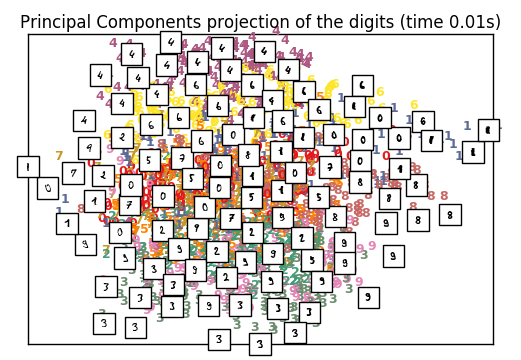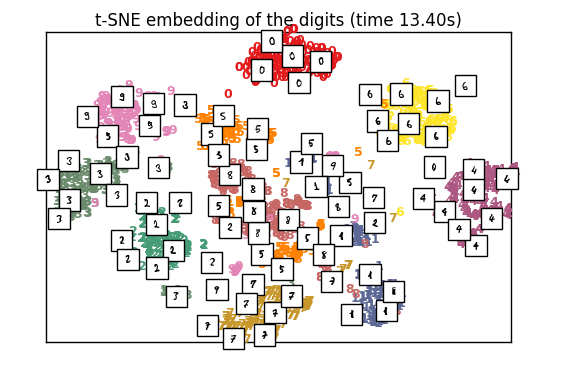## 9. Where and When to use t-SNE?

#### 9.1 Data Scientist

Well for the data scientist the main problem while using t-SNE is the black box type nature of the algorithm. This impedes the process of providing inferences and insights based on the results. Also, another problem with the algorithm is that it doesn’t always provide a similar output on successive runs.

So then how could you use the algorithm? The best way to used the algorithm is to use it for exploratory data analysis. It will give you a very good sense of patterns hidden inside the data. It can also be used as an input parameter for other classification & clustering algorithms.

#### 9.2 Machine Learning Hacker

Reduce the dataset to 2 or 3 dimensions and stack this with a non-linear stacker. Using a holdout set for stacking / blending. Then you can boost the t-SNE vectors using XGboost to get better results.

#### 9.3 Data Science Enthusiasts

For data science enthusiasts who are beginning to work with data science, this algorithm presents the best opportunities in terms of research and performance enhancements. There have been a few research papers attempting to improve the time complexity of the algorithm by utilizing linear functions. But an optimal solution is still required. Research papers on implementing t-SNE for a variety of NLP problems and image processing applications is an unexplored territory and has enough scope.

## 10. Common Fallacies

Following are a few common fallacies to avoid while interpreting the results of t-SNE:

1. For the algorithm to execute properly, the perplexity should be smaller than the number of points. Also, the suggested perplexity is in the range of (5 to 50)
2. Sometimes, different runs with same hyper parameters may produce different results.
3. Cluster sizes in any t-SNE plot must not be evaluated for standard deviation, dispersion or any other similar measures. This is because t-SNE expands denser clusters and contracts sparser clusters to even out cluster sizes. This is one of the reasons for the crisp and clear plots it produces.
4. Distances between clusters may change because global geometry is closely related to optimal perplexity. And in a dataset with many clusters with different number of elements one perplexity cannot optimize distances for all clusters.
5. Patterns may be found in random noise as well, so multiple runs of the algorithm with different sets of hyperparameter must be checked before deciding if a pattern exists in the data.
6. Different cluster shapes may be observed at different perplexity levels.
7. Topology cannot be analyzed based on a single t-SNE plot, multiple plots must be observed before making any assessment.

### Reference

 L.J.P. van der Maaten and G.E. Hinton. Visualizing High-Dimensional Data Using  t-SNE. Journal of Machine Learning Research 9(Nov):2579-2605, 2008

 Jizheng Yi et.al. Facial expression recognition Based on t-SNE and AdaBoostM2.

IEEE International Conference on Green Computing and Communications and IEEE Internet of Things and IEEE Cyber,Physical and Social Computing (2013)

  Walid M. Abdelmoulaa et.al. Data-driven identification of prognostic tumor subpopulations using spatially mapped t-SNE of mass spectrometry imaging data.

12244–12249 | PNAS | October 25, 2016 | vol. 113 | no. 43

  Hendrik Heuer. Text comparison using word vector representations and dimensionality reduction. 8th EUR. CONF. ON PYTHON IN SCIENCE (EUROSCIPY 2015)

### End Notes

Share your experience of working with t-SNE algorithm and if you think its better than PCA. If you have any doubts or questions, feel free to post it in the comments section.

### Learn, compete, hack and get hired

You can also read this article on our Mobile APP•Dr.D.K.Samuel says:

Nice keep them coming
but it says
> Labels train\$label<-as.factor(train\$label)

•dataslee says:

the train data you must have yourself

•Saurabh jugalkishor Jaju says:

Dear Dr Samuel,
The data to execute the R code must be loaded in the R environment. I will provide the link to the dataset. Please download the data, read it in the R environment and then run the code.
Then load the data in R using : read.csv(file.choose()) command in R.

Thankyou for reading the article and please feel free to contact me with anything else relating to the article.

Regards,
Saurabh

•Pete says:

Hi,
This is a great article covering the topic comprehensively.
Would appreciate if you can you share the complete R code.

Looking forward to more articles from you on Advanced topics.

Thanks
Pete

•Mantej Singh Dhanjal says:

Nicely explained. Another great article from Analytics Vidhya. Thank you very much for such a useful information!
I’ll look into that example.

•padhma says:

I simply want to tell you that I’m all new to blogs and truly liked you’re blog site. Very likely I’m likely to bookmark your site .You surely come with remarkable articles. Cheers for sharing your website page.

•Kern Paillard says:

Great article!
I’m curious to know of your thoughts on Kernel PCA…compated to t-SNE.
Essentially, kernel PCA also maps the high dimensions using non-linear methods…

There is a scikit implementation of it here,
http://scikit-learn.org/stable/auto_examples/decomposition/plot_kernel_pca.html

I’ve found performance issues when using Kernel PCA on even relatively medium sized data sets for e.g. ~= 100k rows and had to resort to carrying out kernel pca on chunks of data..

•Donald E. Foss says:

Can you explain the large time delta in the execution in R versus Python? I assume the data set was the same.

PCA
R: 11.360 seconds
Python: 0.01 seconds
tSNE
R: 118.006 seconds
Python: 13.40 seconds

The delta with tSNE is nearly a magnitude, and the delta with PCA is incredible.

•saurabh.jaju2 says:

Actually the datasets are not the same.The point of the code was to show the implementations of tSNE and PCA and compare them in each language. Comparison of performance of python code to R code was not intended .The dataset for R is provided as a link in the article and the dataset for python is loaded sklearn package.

• […] R-Python实现的t-SNE算法综合指南  […]

•samira mzn says:

Hello,
I tested the R code for the t-SNE method and it worked very well, I would also like to know if you have informations on the two dimensionality reduction methods : NeRV and JSE, with the codes R ?

Thanks,

• […] R-Python实现的t-SNE算法综合指南  […]

•Cynthia says:

Great guide – thanks for this!

If you want to check out an interesting use of t-SNE, Displayr recently used it to analyze Middle Eastern politics. Fascinating read: https://www.displayr.com/using-machine-learning-t-sne-to-understand-middle-eastern-politics/

Thanks,
Cynthia

•Shane says:

in order to use this technique for machine learning, the t-SNE model would need to convert future observations (e.g. from a test set), based on the results from the training set

so is it possible for example, to do something like

test_set_transformed <- predict(tsne, newdata = test_set)

where tsne is the model/output from Rtsne() and the training set in your code

•Queen Ohandjo says:

Excellent post.
Code worked well
Now i have to prepare my dataset in this format for a successful run.

Thanks alot.

*crossing fingers* code works on my data

•Queen Ohandjo says:

Hi,
I was able to successfully run the train.csv file.
But when i run my dataset i get the error below
Any help will be much appreciated

> ## calling the installed package
> prostate library(Rtsne)
>
> ## Curating the database for analysis with both t-SNE and PCA
> Labels prostate\$label<-as.factor(prostate\$label)
Error in `\$
> ## for plotting
> colors = rainbow(length(unique(prostate\$label)))
> names(colors) = unique(prostate\$label)
>
> ## Executing the algorithm on curated data
> tsne exeTimeTsne
> ## Plotting
> plot(tsne\$Y, t=’n’, main=”tsne”)
> text(tsne\$Y, labels=prostate\$label, col=colors[prostate\$label])